Test: Maxwell’S Equations

# Test: Maxwell’S Equations - Electrical Engineering (EE)

Test Description

## 20 Questions MCQ Test GATE Electrical Engineering (EE) 2024 Mock Test Series - Test: Maxwell’S Equations

Test: Maxwell’S Equations for Electrical Engineering (EE) 2023 is part of GATE Electrical Engineering (EE) 2024 Mock Test Series preparation. The Test: Maxwell’S Equations questions and answers have been prepared according to the Electrical Engineering (EE) exam syllabus.The Test: Maxwell’S Equations MCQs are made for Electrical Engineering (EE) 2023 Exam. Find important definitions, questions, notes, meanings, examples, exercises, MCQs and online tests for Test: Maxwell’S Equations below.
Solutions of Test: Maxwell’S Equations questions in English are available as part of our GATE Electrical Engineering (EE) 2024 Mock Test Series for Electrical Engineering (EE) & Test: Maxwell’S Equations solutions in Hindi for GATE Electrical Engineering (EE) 2024 Mock Test Series course. Download more important topics, notes, lectures and mock test series for Electrical Engineering (EE) Exam by signing up for free. Attempt Test: Maxwell’S Equations | 20 questions in 60 minutes | Mock test for Electrical Engineering (EE) preparation | Free important questions MCQ to study GATE Electrical Engineering (EE) 2024 Mock Test Series for Electrical Engineering (EE) Exam | Download free PDF with solutions
 1 Crore+ students have signed up on EduRev. Have you?
Test: Maxwell’S Equations - Question 1

### A perfectly conducting filament containing a 250Ω​ resistor is formed into a square as shown in fig.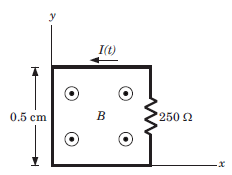​ ​ ​   Que: If B = 6 cos (120πt - 300 )uz T, then the value of I(t) is

Detailed Solution for Test: Maxwell’S Equations - Question 1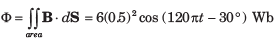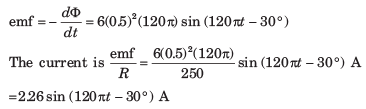Test: Maxwell’S Equations - Question 2

### A perfectly conducting filament containing a 250Ω​ resistor is formed into a square as shown in fig.Que: If B = 2 cosπ(ct - y) uz  μT, where c is the velocity of light, then I(t) is

Detailed Solution for Test: Maxwell’S Equations - Question 2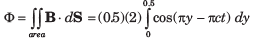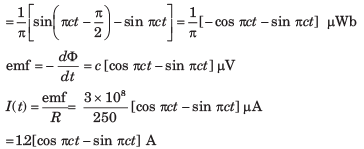Test: Maxwell’S Equations - Question 3

### Consider the fig. The rails have a resistance of 2 Ω/m. The bar moves to the right at a constant speed of 9 m/s in a uniform magnetic field of 0.8 T. The bar is at x = 2 m at t = 0.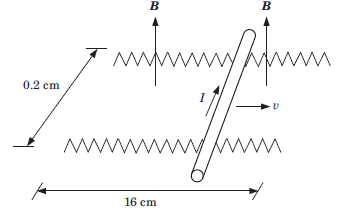Que: If 6 Ω resistor is present across the left-end with the right end open-circuited, then at t = 0.5 sec the current I is

Detailed Solution for Test: Maxwell’S Equations - Question 3

The flux in the left-hand closed loop is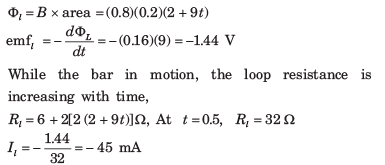Test: Maxwell’S Equations - Question 4

Consider the fig. The rails have a resistance of 2 Ω/m. The bar moves to the right at a constant speed of 9 m/s in a uniform magnetic field of 0.8 T. The bar is at x = 2 m at t = 0.Que: If 6 Ω resistor is present across each end, then I at 0.5 sec is

Detailed Solution for Test: Maxwell’S Equations - Question 4

In this case, there will be contribution to the current from the right loop, which is now closed. The flux in the right loop, whose area decreases with time, is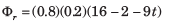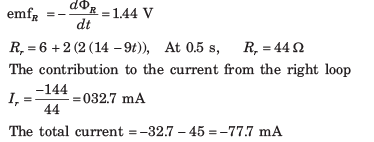Test: Maxwell’S Equations - Question 5

The internal dimension of a coaxial capacitor is a = 1.2 cm, b = 4 cm and c = 40 cm. The homogeneous material inside the capacitor has the parameter ε = 10-11 F/m, μ = 10-5H/m and  σ =10-S/m.The electric field intensity is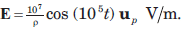Que: The current density J is

Detailed Solution for Test: Maxwell’S Equations - Question 5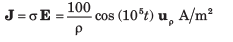Test: Maxwell’S Equations - Question 6

The internal dimension of a coaxial capacitor is a = 1.2 cm, b = 4 cm and c = 40 cm. The homogeneous material inside the capacitor has the parameter ε = 10-11 F/m, μ = 10-5H/m and  σ =10-S/m.The electric field intensity isQue: The quality factor of the capacitor is

Detailed Solution for Test: Maxwell’S Equations - Question 6

Total conduction current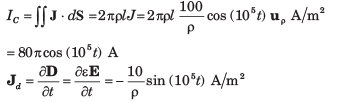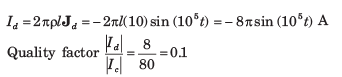Test: Maxwell’S Equations - Question 7

The following fields exist in charge free regions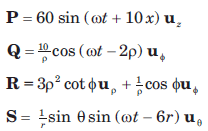The possible electromagnetic fields are

Detailed Solution for Test: Maxwell’S Equations - Question 7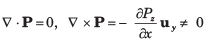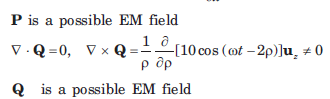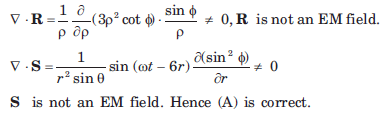Test: Maxwell’S Equations - Question 8

A parallel-plate capacitor with plate area of 5 cmand plate separation of 3 mm has a voltage 50 sin (103 t) V applied to its plates. If εr = 2, the displacement current is

Detailed Solution for Test: Maxwell’S Equations - Question 8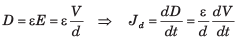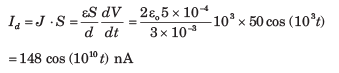Test: Maxwell’S Equations - Question 9

In a coaxial transmission line (εr = 1), the electric field intensity is given by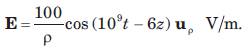The displacement current density is

Detailed Solution for Test: Maxwell’S Equations - Question 9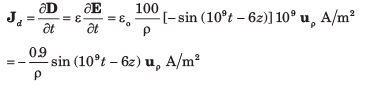Test: Maxwell’S Equations - Question 10

Consider the region defined by |x|,|y| and |z| < 1. Let ε = 5ε0 , μ = 4μo  and σ = 0 the displacement current densityJd  = 20cos(1.5 x 108 t - ax)uy μA/m2. Assume no DC fields are present.

Detailed Solution for Test: Maxwell’S Equations - Question 10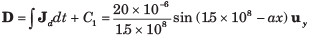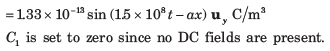Test: Maxwell’S Equations - Question 11

Consider the region defined by |x|,|y| and |z| < 1. Let ε = 5ε0 , μ = 4μo  and σ = 0 the displacement current densityJd  = 20cos(1.5 x 108 t - ax)uy μA/m2. Assume no DC fields are present.

Que: The magnetic field intensity is

Detailed Solution for Test: Maxwell’S Equations - Question 11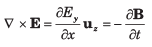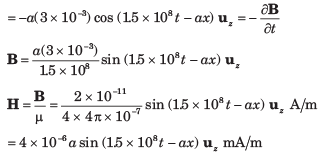Test: Maxwell’S Equations - Question 12

Consider the region defined by |x|,|y| and |z| < 1. Let ε = 5ε0 , μ = 4μo  and σ = 0 the displacement current densityJd  = 20cos(1.5 x 108 t - ax)uy μA/m2. Assume no DC fields are present.

Que: The value of α is

Detailed Solution for Test: Maxwell’S Equations - Question 12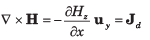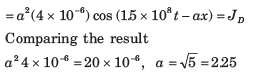Test: Maxwell’S Equations - Question 13

Let  H = 2cos(1010 - βx) uz A/m, μ = 3 x 10-5 H/m, ε = 1.2 x 10-10 F/m and σ = 0 everywhere.

Que: The electric flux density D is

Detailed Solution for Test: Maxwell’S Equations - Question 13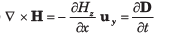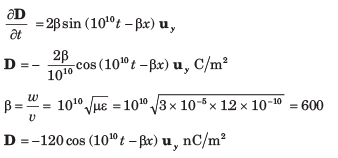Test: Maxwell’S Equations - Question 14

Let  H = 2cos(1010 - βx) uz A/m, μ = 3 x 10-5 H/m, ε = 1.2 x 10-10 F/m and σ = 0 everywhere.

Que: The magnetic flux density B is

Detailed Solution for Test: Maxwell’S Equations - Question 14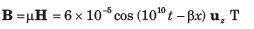Test: Maxwell’S Equations - Question 15

A material has  σ = 0 and εr = 1. The magnetic field intensity is H = 4cos ( 106t  - 0.01z)μy A/m.

Que: The electric field intensity E is

Detailed Solution for Test: Maxwell’S Equations - Question 15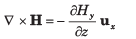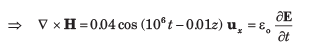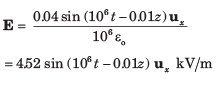Test: Maxwell’S Equations - Question 16

A material has  σ = 0 and εr = 1. The magnetic field intensity is H = 4cos ( 106t  - 0.01z)μy A/m.

Que: The value of  μr is

Detailed Solution for Test: Maxwell’S Equations - Question 16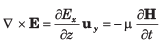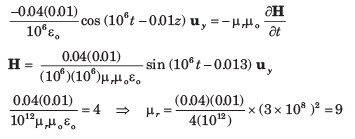Test: Maxwell’S Equations - Question 17

The surface ρ = 3 and 10 mm, and z = 0 and 25 cm are perfect conductors. The region enclosed by these surface has μ = 25 x 10-6  H/m, ε = 4 x 10-11 F/m and σ = 0. If H = 2/ρ cos8πz cosωt uø A/m,  then the value of ω is

Detailed Solution for Test: Maxwell’S Equations - Question 17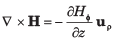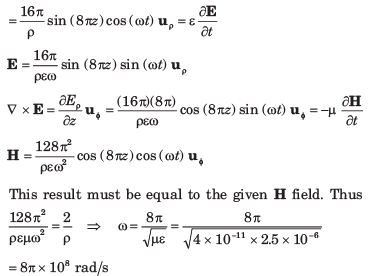Test: Maxwell’S Equations - Question 18

For distilled water μ = μo , ε = 81εo and  σ = 2 x 10-3 S/m, the ratio of conduction current density to displacement current density at 1 GHz is

Detailed Solution for Test: Maxwell’S Equations - Question 18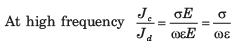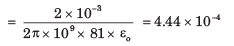Test: Maxwell’S Equations - Question 19

A conductor with cross-sectional area of 10 cmcarrier a conductor current 2sin (109 t) mA. If σ = 2.5 x 106 S/m and εr 4.6 the magnitude of the displacement current density is

Detailed Solution for Test: Maxwell’S Equations - Question 19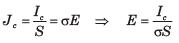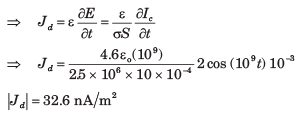Test: Maxwell’S Equations - Question 20

In a certain region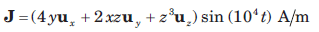If volume charge density ρv in z = 0 plane is zero, then ρv is

Detailed Solution for Test: Maxwell’S Equations - Question 20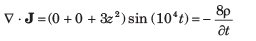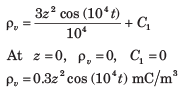## GATE Electrical Engineering (EE) 2024 Mock Test Series

23 docs|285 tests
Information about Test: Maxwell’S Equations Page
In this test you can find the Exam questions for Test: Maxwell’S Equations solved & explained in the simplest way possible. Besides giving Questions and answers for Test: Maxwell’S Equations, EduRev gives you an ample number of Online tests for practice

## GATE Electrical Engineering (EE) 2024 Mock Test Series

23 docs|285 tests